Foxtable(狐表)用户栏目专家坐堂 → 求助：窗体有表格，查找没反应或无法显示定位

共有383人关注过本帖树形打印复制链接

# 主题：求助：窗体有表格，查找没反应或无法显示定位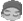zhyqs
1楼 | 信息 | 搜索 | 邮箱 | 主页 | UC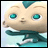加好友发短信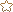Dim rm As WinForm.TextBox = e.Form.Controls("TextBox1")Dim str As String = rm.valueDim dr As DataRow = DataTables("脉络").Find("名 = '" & str & "'")If dr IsNot Nothing Then    Dim idr As String = Tables("脉络").FindRow(dr)    If idr >= 0 Then        Tables("脉络").Position = idr '定位该行    End IfEnd If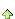2楼 | 信息 | 搜索 | 邮箱 | 主页 | UC加好友发短信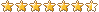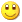Post By：2020/2/14 21:08:00 [只看该作者]

 窗口表是副本？Dim rm As WinForm.TextBox = e.Form.Controls("TextBox1")Dim str As String = rm.valueDim idr As integer = Tables("脉络").FindRow("名 = '" & str & "'")If idr >= 0 Then        Tables("窗口名称_table1").Position = idr '定位该行End Ifzhyqs
3楼 | 信息 | 搜索 | 邮箱 | 主页 | UC加好友发短信Post By：2020/2/14 21:35:00 [只看该作者]

 谢谢版主回复！窗口表不是副本，已知单元格内容为德全两个字，查找全，没反应，查找德全，提示该table的名称不存在Dim rm As WinForm.TextBox = e.Form.Controls("TextBox1")Dim str As String = rm.valueDim idr As Integer = Tables("脉络").FindRow("名 = '" & str & "'")If idr >= 0 Then        Tables("脉络查录_table1").Position = idr '定位该行End If [此贴子已经被作者于2020/2/14 21:42:21编辑过]4楼 | 信息 | 搜索 | 邮箱 | 主页 | UC加好友发短信Post By：2020/2/14 21:38:00 [只看该作者]

 Dim rm As WinForm.TextBox = e.Form.Controls("TextBox1")Dim str As String = rm.valueDim idr As Integer = Tables("脉络").FindRow("名 like '%" & str & "%'")If idr >= 0 Then        Tables("脉络").Position = idr '定位该行End Ifzhyqs
5楼 | 信息 | 搜索 | 邮箱 | 主页 | UC加好友发短信Post By：2020/2/14 21:48:00 [只看该作者]

 非常感谢！后面这个代码成功了，之前我也加了LIKE 也不行，我来仔细看看